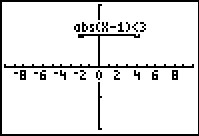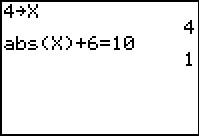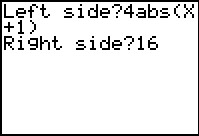# Activities

••• ##### Subject Area

• Math: Algebra I: Absolute Value Functions
• Math: Algebra I: Equations and Inequalities

• ##### Author9-12

60 Minutes

• ##### Device
• TI-83 Plus Family
• TI-84 Plus
• TI-84 Plus Silver Edition

TI Connect™

• ##### Accessories

TI Connectivity Cable

• ##### Report an Issue

Absolutely!#### Activity Overview

Students solve linear absolute value equations in a single variable and use their graphing calculator to verify solutions.

#### Key Steps

•In the first problem, students will solve absolute value linear inequalities and check the solutions using the Test menu on the graphing calculator.

•In the second problem, students use the ABSINEQ program to graph absolute value linear inequalities and are introduced to writing compound inequalities.

•In the third problem, students learn how to simplify absolute value inequalities before writing it as a compound inequality. They will verify their answers by graphing the inequality using the ABSINEQ program.